Z9-I-4

Kate thought a five-digit integer. She wrote the sum of this number and its half at the first line to the workbook. On the second line wrote a total of this number and its one fifth. On the third row she wrote a sum of this number and its one nines. Finally, all three lines sum and result wrote on the fourth line. Then she was amazing found that on the fourth line has writed cube of certain natural number.

Determine the smallest number Kate can think in the beginning.

Result

n =  11250

Solution:Leave us a comment of example and its solution (i.e. if it is still somewhat unclear...):Be the first to comment!To solve this verbal math problem are needed these knowledge from mathematics:

Do you solve Diofant problems and looking for a calculator of Diofant integer equations?

Next similar examples:

1. One hundred stamps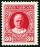A hundred letter stamps cost a hundred crowns. Its costs are four levels - twenty tenths , one crown, two-crown and five-crown. How many are each type of stamps? How many does the problem have solutions?
2. Last digitWhat is the last number of 2016 power of 2017
3. Three-digitHow many three-digit natural numbers do not have the number 7?
4. Six-digit primesFind all six-digit prime numbers that contain each one of digits 1,2,4,5,7 and 8 just once. How many are they?
5. RemainderA is an arbitrary integer that gives remainder 1 in the division with 6. B is an arbitrary integer that gives remainder 2 the division by. What makes remainder in division by 3 product of numbers A x B ?
6. Cakes Z8-I-5Mom brought 10 cakes of three types: kokosek was less than laskonek and most were caramel cubes. John chose two different kinds of cakes, Stephan did the same and for Margerith leave only the cakes of the same type. How many kokosek, laskonek and caramel cIn six baskets, the seller has fruit. In individual baskets, there are only apples or just pears with the following number of fruits: 5,6,12,14,23 and 29. "If I sell this basket," the salesman thinks, "then I will have just as many apples as a pear." Which
8. Unknown numberUnknown number is divisible by exactly three different primes. When we compare these primes in ascending order, the following applies: • Difference first and second prime number is half the difference between the third and second prime numbers. • The produ
9. Tunnels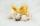Mice had built an underground house consisting of chambers and tunnels: • each tunnel leading from the chamber to the chamber (none is blind) • from each chamber lead just three tunnels into three distinct chambers, • from each chamber mice can get to any
10. Skiing meeting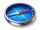On the skiing meeting came four friends from 4 world directions and led the next interview. Charles: "I did not come from the north or from the south." Mojmir "But I came from the south." Joseph: "I came from the north." Zdeno: "I come from the south." W
11. DivisorsThe sum of all divisors unknown odd number is 2112. Determine sum of all divisors of number which is twice of unknown numbers.
12. Pyramid Z8–I–6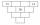Each brick of pyramid contains one number. Whenever possible, the number in each brick is lowest common multiple of two numbers of bricks lying directly above it. That number may be in the lowest brick? Determine all possibilities.
13. Toy carsPavel has a collection of toy cars. He wanted to regroup them. But in the division of three, four, six, and eight, he was always one left. Only when he formed groups of seven, he divided everyone. How many toy cars have in the collection?
14. Z9–I–4 MO 2017Numbers 1, 2, 3, 4, 5, 6, 7, 8 and 9 were prepared for a train journey with three wagons. They wanted to sit out so that three numbers were seated in each carriage and the largest of each of the three was equal to the sum of the remaining two. The conducto
15. Cube root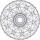Find cube root of 18
16. Cows and calves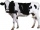There are 168 cows and calves in the cowshed. Cows are in nine stalls and calves in four stalls. Same count cows are in every cow stall and three more in each calf stall than in a cow stall. What is the capacity of the stalls for cows and what for calves?
17. Pet store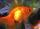In a pet store, they are selling out the fish from one aquarium. Ondra wanted half of all fish, but they don't wish cut by hal fany fish he got one more than demanded. Matthew wished the remaining half of the fish, but as Andrew got half the fish more th